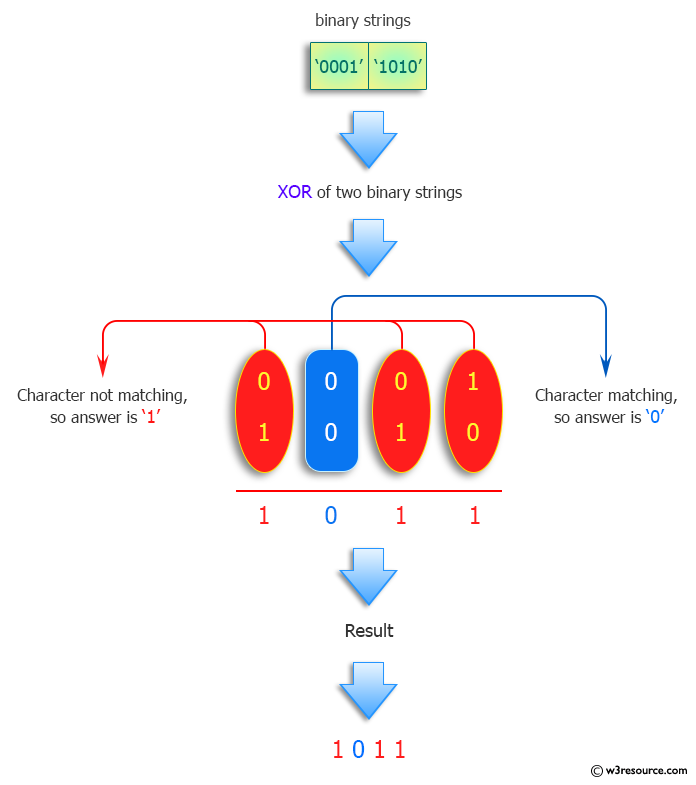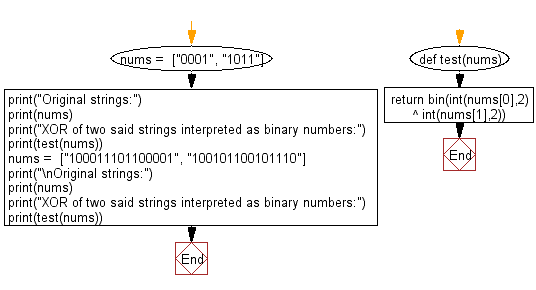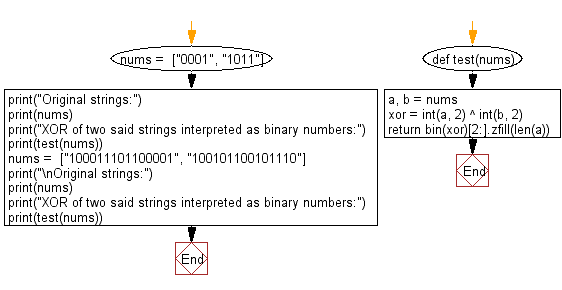﻿ Python: Find the XOR of two given strings interpreted as binary numbers - w3resource# Python: Find the XOR of two given strings interpreted as binary numbers

## Python Programming Puzzles: Exercise-25 with Solution

Write a Python program to find the XOR of two given strings interpreted as binary numbers.

Note: XOR represents the inequality function, i.e., the output is true if the inputs are not alike otherwise the output is false. A way to remember XOR is "must have one or the other but not both". XOR can also be viewed as addition modulo 2. As a result, XOR gates are used to implement binary addition in computers.

```Input:
['0001', '1011']
Output:
0b1010

Input:
['100011101100001', '100101100101110']
Output:
0b110001001111
```

Pictorial Presentation:Sample Solution-1:

Python Code:

``````#License: https://bit.ly/3oLErEI
def test(nums):
return bin(int(nums,2) ^ int(nums,2))
nums =  ["0001", "1011"]
print("Original strings:")
print(nums)
print("XOR of two said strings interpreted as binary numbers:")
print(test(nums))
nums =  ["100011101100001", "100101100101110"]
print("\nOriginal strings:")
print(nums)
print("XOR of two said strings interpreted as binary numbers:")
print(test(nums))
``````

Sample Output:

```Original strings:
['0001', '1011']
XOR of two said strings interpreted as binary numbers:
0b1010

Original strings:
['100011101100001', '100101100101110']
XOR of two said strings interpreted as binary numbers:
0b110001001111
```

Flowchart:## Visualize Python code execution:

The following tool visualize what the computer is doing step-by-step as it executes the said program:

Sample Solution-2:

Python Code:

``````#License: https://bit.ly/3oLErEI

def test(nums):
a, b = nums
xor = int(a, 2) ^ int(b, 2)
return bin(xor)[2:].zfill(len(a))

nums =  ["0001", "1011"]
print("Original strings:")
print(nums)
print("XOR of two said strings interpreted as binary numbers:")
print(test(nums))
nums =  ["100011101100001", "100101100101110"]
print("\nOriginal strings:")
print(nums)
print("XOR of two said strings interpreted as binary numbers:")
print(test(nums))
``````

Sample Output:

```Original strings:
['0001', '1011']
XOR of two said strings interpreted as binary numbers:
1010

Original strings:
['100011101100001', '100101100101110']
XOR of two said strings interpreted as binary numbers:
000110001001111
```

Flowchart:## Visualize Python code execution:

The following tool visualize what the computer is doing step-by-step as it executes the said program:

Python Code Editor :

Have another way to solve this solution? Contribute your code (and comments) through Disqus.

What is the difficulty level of this exercise?

Test your Programming skills with w3resource's quiz.

﻿

## Python: Tips of the Day

Clamps num within the inclusive range specified by the boundary values x and y:

Example:

```def tips_clamp_num(num,x,y):
return max(min(num, max(x, y)), min(x, y))
print(tips_clamp_num(2, 4, 6))
print(tips_clamp_num(1, -1, -6))
```

Output:

```4
-1
```